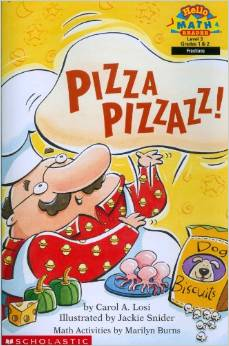# Fourths

6 teachers like this lesson
Print Lesson

## Objective

SWBAT partition circles and rectangles into four equal shares.

#### Big Idea

Let’s Share! In this lesson students will learn to divide circles and rectangles into four equal shares and describe the shares using the vocabulary fourths and quarters.

## Activating Strategy

10 minutes

I like to start this lesson with reading the book, “Pizza Pizzazz!The students really respond well to this book, and they can easily relate to pizza. Since food is a natural way for kids to relate real world examples, this book keeps their attention. The story begins with 2 people sharing a pizza and continues until there are 8 people sharing a pizza.

After reading the story, I demonstrate how to use one of the student cut outs and fold it into fourths.  Then I hand out the Student cutouts and have students cut them and fold them into fourths.

I really like bringing in food examples because being able to recognize equal parts or equal shares will help children understand real-life situations in which words such as half, fourth, or quarter are used. I frequently point out that these words are connected to many real-life uses, such as time (half-past ten), distances (a quarter mile), dozens (a half dozen), and money (a quarter). In turn, such informal real-life applications will help children see that the work they do with shapes is related to things that people do every day.

## Teaching Strategies

15 minutes

After discussing fourths, I display the first slide of the PowerPoint Fourths.  Then I read the following aloud:

Two friends will share a pizza. Then two more friends come. Now four friends will share the pizza. How can the pizza be cut so each friend gets an equal share? How many equal shares are there?

I guide children to share the pizza for two friends first by asking these questions:

• How can you share the pizza for two friends to have equal shares? (I could draw a line to make 2 parts that are the same size.)
• What is each part called? (a half)

I then have a student draw a line to show halves.

• How can you change your drawing so that four friends can each have an equal share? (I can draw another line to make 4 equal parts.)

I then have another student draw a second line to show fourths.

• Who gets a smaller equal share, two friends who share a pizza or four friends who share a pizza? (Four friends get a smaller share because their equal shares are smaller than halves.)

## Independent Practice

30 minutes

Then I display the next slide on the PPT.  I work through the model with children, emphasizing the different names for the equal shares.

• What are two different names for 4 equal parts? (fourths or quarters)
• How many fourths make a whole? (4)
• How many quarters make a whole? (4)

Once I’m sure that students understand that a fourth and a quarter of the shape is one equal part of the whole, I release them to continue the Fourths_worksheet independently.

In this video, the student successfully demonstrates the understanding that fourths need to be equal in size.

## Closing/Summarizing

5 minutes

To close out the lesson, I have students use their journal to draw two squares. Then I ask them to draw lines to show fourths and color a fourth of the first square. Then I say to color the second square to show a whole.